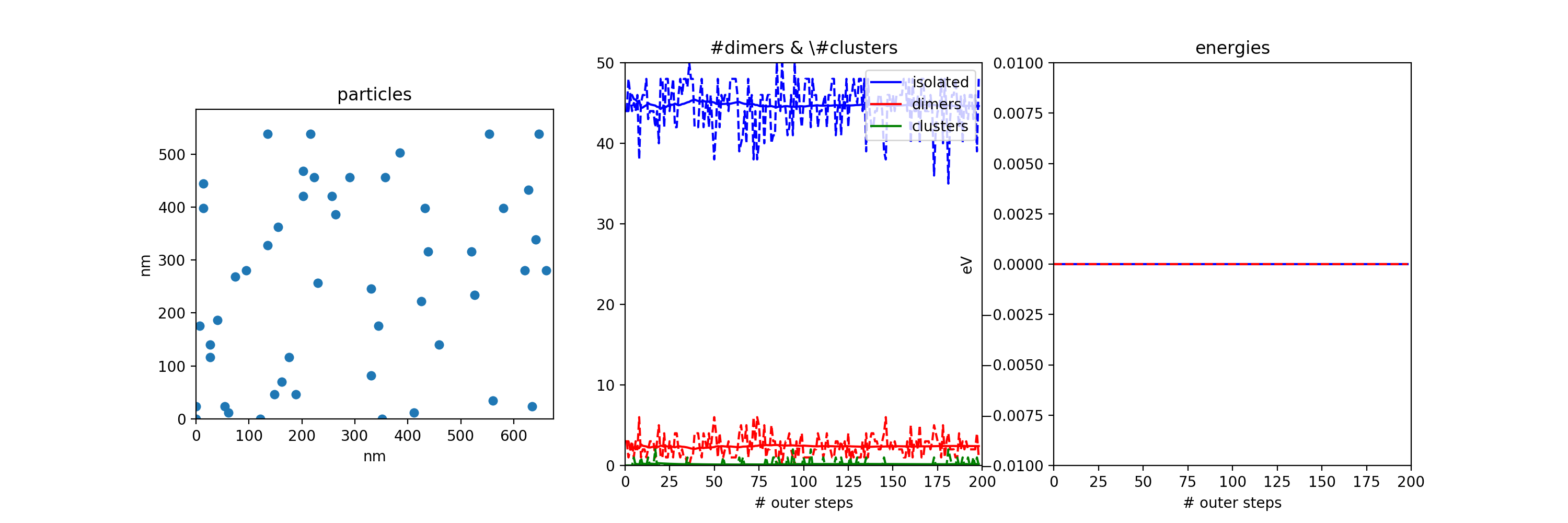#CP2K Open Source Molecular Dynamics

### Sidebar

#### For Developers

exercises:2017_ethz_mmm:mc_and_kmc

This is an old revision of the document!

# Monte Carlo simulations for the estimation of molecule pair interatcion

In this exercise you will perform a MC simulation for different coverages of “sumanene” mlecules adsorbed on a Ag(111) substrate hypothesizing different posisble values for the nearest neighbor energy in the molecule-molecule interaction

All files of this exercise be downloaded from the wiki: e3_bis.zip Download the exercise into your $HOME folder and unzip it. you@eulerX ~$ wget http://www.cp2k.org/_media/exercises:2017_ethz_mmm:e3_bis.zip
you@eulerX ~$unzip exercises:2017_ethz_mmm:e3_bis.zip you@eulerX ~$ cd exercise_3

In an experiment performed at Empa, sumanene molecules were adsorbed on a Ag(111) surface. It was found that at very low coverage (0.02) 30% of the molecules were weakly bound into dimers. [ http://dx.doi.org/10.1021/ja504126z J. Am. Chem. Soc. 2014, 136, 13666−13671]

The python code mc.py mimics the Ag(111) substrate as a rigid hexagonal lattice onto which molecules (blue dots) are allowed to move by random discrete steps

While in execution, the code will show you snapshots of the positions of the molecules on the lattice on the left panel.In the central panel average values for the number of isolated molecules, the number of dimers and the number of clusters is plotted. On the right panel the average energy of the system is plotted. Intermediate average values are also written in a output file data_de_T.out where de and T are the input values of the dimer energy and of the Temperature also a final snapshot of the graphic panels is saved as png image

TASK 1 Execute the program with parameters:
coverage 0.02
number of cycles 200
binding energy in eV 0.0
Temperature in K 200

Estimate the dimer binding energy as DE=kT * ln(n0/nexp) where k is Boltzmann's constant T is the simulation (and experiment) temperature n0 is teh concentration of dimers in the case of zero interaction nexp is the concentration of dimers found in the experiment

TASK2 repeat the simulation using as DE your estimate. What do you get?

TASK3 Repeat the simulation with coverage 0.1 and DE=-0.02 and DE=-0.1 Describe what you obtain. Now try coverage=0.1 T=400 DE=-0.1 Comment the result

TASK4 have a look at the pyhton code, identify the main MC steps in the MAIN part of the code

#### MAIN MC LOOP

and also the section

#### DECIDE whether to accept or not the move

What do you think it would happen if you replace the condition

if np.random.random()<np.exp(-beta*deltae)

with the condition

if enew<eold
The most complex (and unefficient) sections of the code are the two functions
def allconnected(m,id,nx,ny)

which finds out all teh molecules that are connected to a given one

def neighbors(a,id,nx,ny)

which finds out all the molecules that are 1st neighbors to a given one.

The function “allconnected” is quite intuitive and inefficient. Can you imagine roughly a more efficient function to perform the same task?

The code, at each step, moves a particle chosen randomly to a new site chosen randomly. Would it be correct to move all particles in a step? what would change?
exercises/2017_ethz_mmm/mc_and_kmc.1489071927.txt.gz · Last modified: 2020/08/21 10:15 (external edit)

### Page Tools